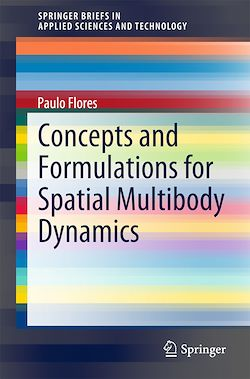# Concepts and Formulations for Spatial Multibody Dynamics

-English
83 Pages

Description

This book will be particularly useful to those interested in multibody simulation (MBS) and the formulation for the dynamics of spatial multibody systems. The main types of coordinates that can be used in the formulation of the equations of motion of constrained multibody systems are described. The multibody system, made of interconnected bodies that undergo large displacements and rotations, is fully defined.
Readers will discover how Cartesian coordinates and Euler parameters are utilized and are the supporting structure for all methodologies and dynamic analysis, developed within the multibody systems methodologies. The work also covers the constraint equations associated with the basic kinematic joints, as well as those related to the constraints between two vectors.
The formulation of multibody systems adopted here uses the generalized coordinates and the Newton-Euler approach to derive the equations of motion. This formulation results in the establishment of a mixed set of differential and algebraic equations, which are solved in order to predict the dynamic behavior of multibody systems. This approach is very straightforward in terms of assembling the equations of motion and providing all joint reaction forces.
The demonstrative examples and discussions of applications are particularly valuable aspects of this book, which builds the reader’s understanding of fundamental concepts.

Subjects

##### Automation

Informations